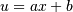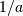# numpy.polynomial.hermite_e.hermeint¶

`numpy.polynomial.hermite_e.``hermeint`(c, m=1, k=[], lbnd=0, scl=1, axis=0)[source]

Integrate a Hermite_e series.

Returns the Hermite_e series coefficients c integrated m times from lbnd along axis. At each iteration the resulting series is multiplied by scl and an integration constant, k, is added. The scaling factor is for use in a linear change of variable. (“Buyer beware”: note that, depending on what one is doing, one may want scl to be the reciprocal of what one might expect; for more information, see the Notes section below.) The argument c is an array of coefficients from low to high degree along each axis, e.g., [1,2,3] represents the series `H_0 + 2*H_1 + 3*H_2` while [[1,2],[1,2]] represents ```1*H_0(x)*H_0(y) + 1*H_1(x)*H_0(y) + 2*H_0(x)*H_1(y) + 2*H_1(x)*H_1(y)``` if axis=0 is `x` and axis=1 is `y`.

Parameters: c : array_like Array of Hermite_e series coefficients. If c is multidimensional the different axis correspond to different variables with the degree in each axis given by the corresponding index. m : int, optional Order of integration, must be positive. (Default: 1) k : {[], list, scalar}, optional Integration constant(s). The value of the first integral at `lbnd` is the first value in the list, the value of the second integral at `lbnd` is the second value, etc. If `k == []` (the default), all constants are set to zero. If `m == 1`, a single scalar can be given instead of a list. lbnd : scalar, optional The lower bound of the integral. (Default: 0) scl : scalar, optional Following each integration the result is multiplied by scl before the integration constant is added. (Default: 1) axis : int, optional Axis over which the integral is taken. (Default: 0). New in version 1.7.0. S : ndarray Hermite_e series coefficients of the integral. ValueError If `m < 0`, `len(k) > m`, `np.isscalar(lbnd) == False`, or `np.isscalar(scl) == False`.

Notes

Note that the result of each integration is multiplied by scl. Why is this important to note? Say one is making a linear change of variablein an integral relative to x. Then .. math::dx = du/a, so one will need to set scl equal to- perhaps not what one would have first thought.

Also note that, in general, the result of integrating a C-series needs to be “reprojected” onto the C-series basis set. Thus, typically, the result of this function is “unintuitive,” albeit correct; see Examples section below.

Examples

```>>> from numpy.polynomial.hermite_e import hermeint
>>> hermeint([1, 2, 3]) # integrate once, value 0 at 0.
array([ 1.,  1.,  1.,  1.])
>>> hermeint([1, 2, 3], m=2) # integrate twice, value & deriv 0 at 0
array([-0.25      ,  1.        ,  0.5       ,  0.33333333,  0.25      ])
>>> hermeint([1, 2, 3], k=1) # integrate once, value 1 at 0.
array([ 2.,  1.,  1.,  1.])
>>> hermeint([1, 2, 3], lbnd=-1) # integrate once, value 0 at -1
array([-1.,  1.,  1.,  1.])
>>> hermeint([1, 2, 3], m=2, k=[1, 2], lbnd=-1)
array([ 1.83333333,  0.        ,  0.5       ,  0.33333333,  0.25      ])
```

#### Previous topic

numpy.polynomial.hermite_e.hermeder